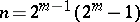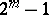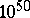# Perfect number

(diff) ← Older revision | Latest revision (diff) | Newer revision → (diff)

A positive integer with the property that it coincides with the sum of all its positive divisors other than the number itself.

Thus, an integeris a perfect number ifFor example, the numbers 6, 28, 496, 8128, 33550336are perfect.

Perfect numbers are closely connected with Mersenne primes (see Mersenne number), i.e. prime numbers of the form. Euclid already established that the numberis perfect ifis a prime number. L. Euler showed that these exhaust all even perfect numbers.

It is still (1983) unknown whether the set of even perfect numbers is finite or infinite, that is, it is unknown whether the set of Mersenne primesis finite or not. It is also unknown whether or not there are any odd perfect numbers.

Twenty-seven even perfect numbers have been found up till now (1983). The first twenty-three of them correspond to the following values of: 2, 3, 5, 7, 13, 17, 31, 61, 89, 107, 127, 521, 607, 1279, 2203, 2281, 3217, 4253, 4423, 9689, 9941, and 11213. A list of perfect numbers from the twelfth to the twenty-fourth is given in . The even numbers from the twenty-fifth to the twenty-seventh correspond to the following values of: 21701, 23209 and 44497 (see ). It has been shown (see ) that there are no odd perfect numbers in the interval from 1 to.

How to Cite This Entry:
Perfect number. Encyclopedia of Mathematics. URL: http://encyclopediaofmath.org/index.php?title=Perfect_number&oldid=15832
This article was adapted from an original article by S.A. Stepanov (originator), which appeared in Encyclopedia of Mathematics - ISBN 1402006098. See original article